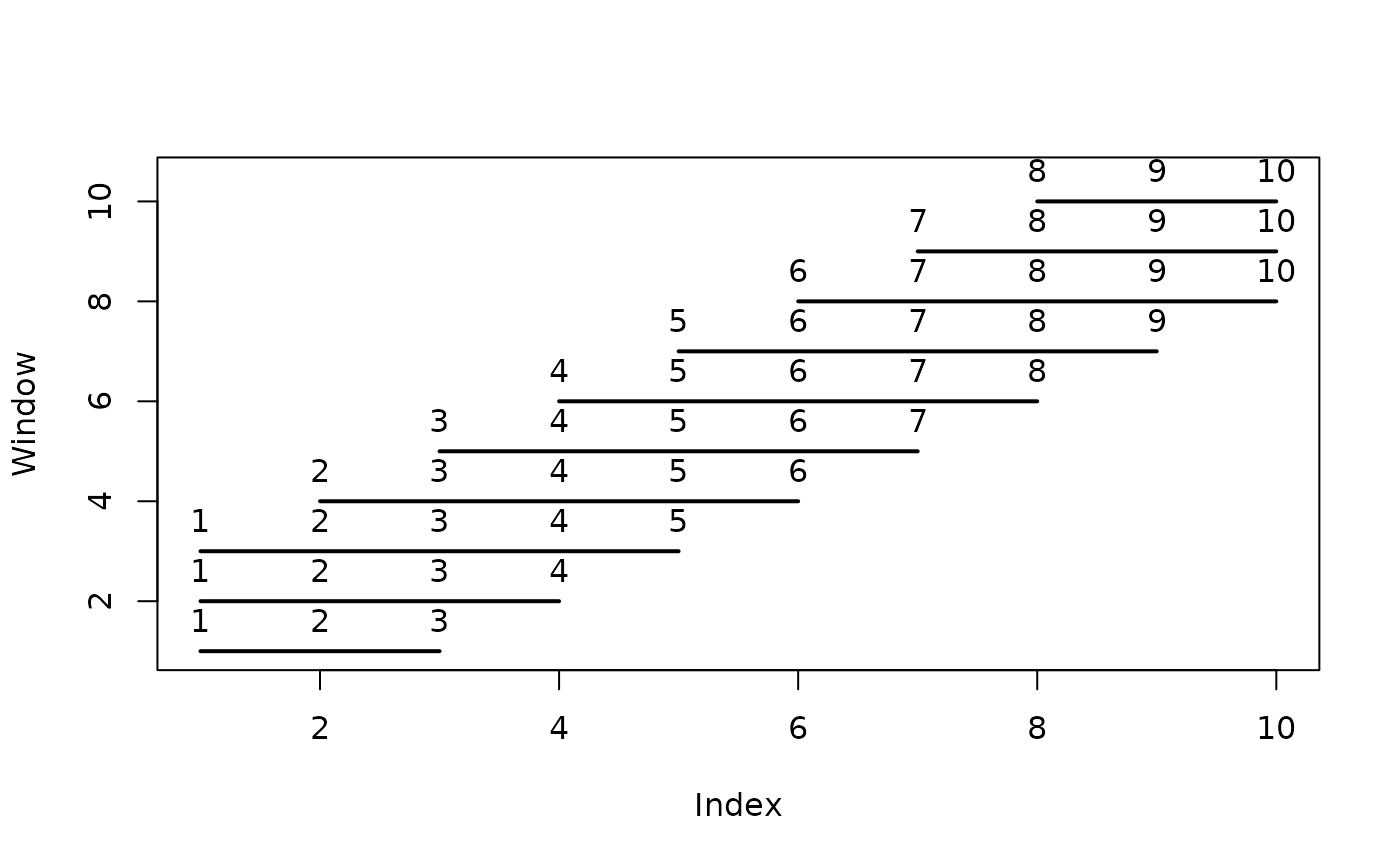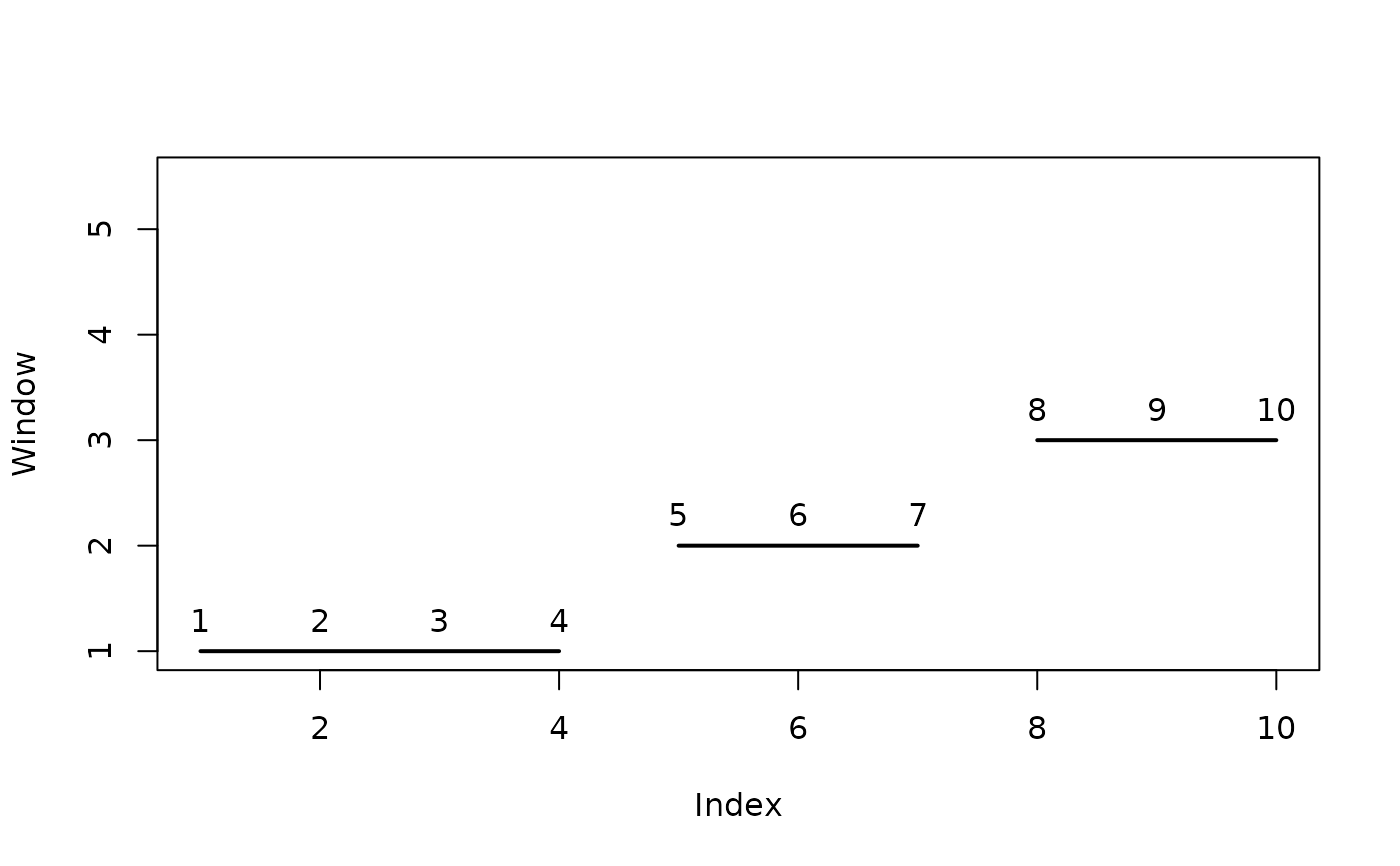There will be $$(m - 1) / 2$$ points both at the beginning and at the end of the data series for which a complete $$m$$-width window cannot be obtained. To prevent data loss, progressively wider/narrower windows are evaluated at both ends of the data series.

## Usage

window_sliding(n, m, ...)

# S4 method for integer,integer
window_sliding(n, m, i = NULL)

# S4 method for numeric,numeric
window_sliding(n, m, i = NULL)

## Arguments

n

An integer giving the length of the data series (will be coerced with as.integer() and hence truncated toward zero).

m

An odd integer giving the window size, i.e. the number of adjacent points to be used (will be coerced with as.integer() and hence truncated toward zero).

...

Currently not used.

i

A vector integer specifying the indices of the data points for which windows should be returned. If NULL (the default), windows are evaluated for each data point.

## Value

Returns a length-$$n$$

list of integer vectors (indices of the data points in each window).

Other moving windows: window_tumbling()

N. Frerebeau

## Examples

## Length of the data series
n <- 10

## Progressive sliding windows
sliding <- window_sliding(n = n, m = 5)

plot(NULL, xlim = c(1, n), ylim = c(1, 10.5), xlab = "Index", ylab = "Window")
for (i in seq_along(sliding)) {
w <- sliding[[i]]
text(x = w, y = rep(i, length(w)), labels = w, pos = 3)
lines(w, rep(i, length(w)), type = "l", lwd = 2)
}## Tumbling windows
## (compare with drop = TRUE)
tumbling <- window_tumbling(n = n, m = 3, drop = FALSE)

plot(NULL, xlim = c(1, n), ylim = c(1, 5.5), xlab = "Index", ylab = "Window")
for (i in seq_along(tumbling)) {
w <- tumbling[[i]]
text(x = w, y = rep(i, length(w)), labels = w, pos = 3)
lines(w, rep(i, length(w)), type = "l", lwd = 2)
}